Finding Perimeter

 Problem Set 1 Problem Set 2 Problem Set 3Select a problem set using the buttons above, then use your mouse or tab key to select a question. Fill in the blank with the correct answer for each problem. When you have answered all of the questions, ask Charlie how you did.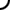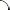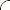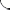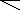For questions 1 through 3 use the diagram below.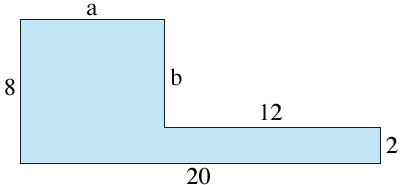The length of the side labeled "a" is The length of the side labeled "b" is The perimeter of the figure is   For questions 4 through 6 use the diagram below.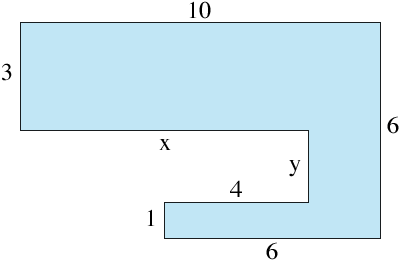The length of the side labeled "x" is The length of the side labeled "y" is The perimeter of the figure is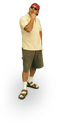For questions 1 through 3 use the diagram below.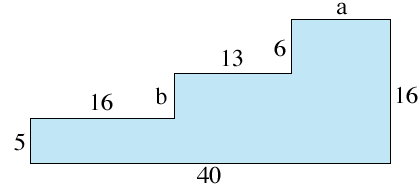The length of the side labeled "a" is The length of the side labeled "b" is The perimeter of the figure is   For questions 4 through 6 use the diagram below.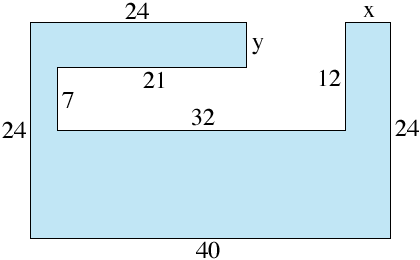The length of the side labeled "x" is The length of the side labeled "y" is The perimeter of the figure isFor questions 1 through 3 use the diagram below.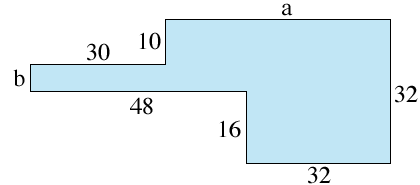The length of the side labeled "a" is The length of the side labeled "b" is The perimeter of the figure is   For questions 4 through 6 use the diagram below.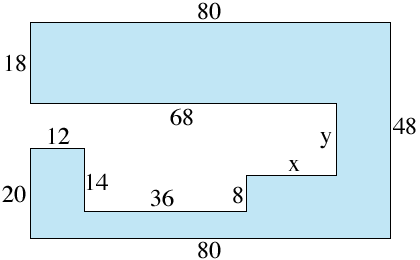The length of the side labeled "x" is The length of the side labeled "y" is The perimeter of the figure is©  2009  LarryPerezWeb Design:  Patrick Quigley